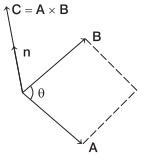# Vector or Cross Product of Two Vectors in Physics – Scalars and Vectors

Vector Product of Two Vectors a and b is:
The vector product of two vectors is equal to the product of their magnitudes and the sine of the smaller angle between them. It is denoted by x (cross).
A x B = AB sin θ $$\hat{\mathbf{n}}$$We are giving a detailed and clear sheet on all Physics Notes that are very useful to understand the Basic Physics Concepts.

## Vector or Cross Product of Two Vectors in Physics – Scalars and Vectors

Vector Cross Product Properties
(i) Vector product is not commutative, i.e.
A x BB x A [∴ (A x B) = – (B x A)]

(ii) Vector product is distributive, i.e.
A x (B + C)= A x B + A x C

(iii) Vector product of two parallel vectors is zero, i.e.
A x B = AB sin 0° = 0

(iv) Vector product of any vector with itself is zero.
A x A = AA sin 0° = 0

(v) Vector product of orthogonal unit vectors
$$\hat{\mathbf{i}} \times \hat{\mathbf{i}}=\hat{\mathbf{j}} \times \hat{\mathbf{j}}=\hat{\mathbf{k}} \times \hat{\mathbf{k}}=0$$

and $$\hat{\mathbf{i}} \times \hat{\mathbf{j}}=-\hat{\mathbf{j}} \times \hat{\mathbf{i}}=\hat{\mathbf{k}}$$
$$\hat{\mathbf{j}} \times \hat{\mathbf{k}}=-\hat{\mathbf{k}} \times \hat{\mathbf{j}}=\hat{\mathbf{i}}$$
$$\hat{\mathbf{k}} \times \hat{\mathbf{i}}=-\hat{\mathbf{i}} \times \hat{\mathbf{k}}=\hat{\mathbf{j}}$$(vi) Vector product in cartesian coordinates
$$\mathbf{A} \times \mathbf{B}=\left(A_{x} \hat{\mathbf{i}}+A_{y} \hat{\mathbf{j}}+A_{z} \hat{\mathbf{k}}\right) \times\left(B_{x} \hat{\mathbf{i}}+B_{y} \hat{\mathbf{j}}+B_{z} \hat{\mathbf{k}}\right)$$= (AyBy – AzBy)$$\hat{\mathbf{i}}$$ – (AxBz – BxAz)$$\hat{\mathbf{j}}$$ + (AxBy – AyBx)$$\hat{\mathbf{k}}$$

Direction of Vector Cross Product
When C = A x B, the direction of C is at right angles to the plane containing the vectors A and B. The direction is determined by the right hand screw rule and right hand thumb rule.(i) Right Hand Screw Rule:
Rotate a right handed screw from first vector (A) towards second vector (B). The direction in which the right handed screw moves gives the direction of vector (C).

(ii) Right Hand Thumb Rule:
Curl the fingers of your right hand from A to B. Then, the direction of the erect thumb will point in the direction of A x B.

Scalars and Vectors Topics: